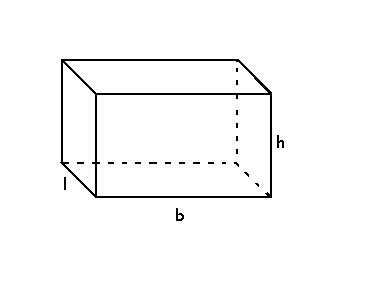# Volume of a Rectangular Prism Formula

The volume of a rectangular prism formula is obtained by multiplying the length, breadth and height of the prism. To recall, a prism that has 2 parallel rectangular bases and 4 rectangular faces is a rectangular prism. The mathematical literature suggests to any polyhedron like this as a Cuboid. It has 6 flat rectangular faces and all the angles are right-angled. The other names of such rectangular prism are rectangular hexahedron, rectangular parallelepiped and right rectangular prism.The volume of a Rectangular Prism Formula = lbh

## Formula for Volume of a Rectangular Prism

The Volume of a Rectangular Prism Formula is,

 Volume of a Rectangular Prism (V) = l × b × h

Where,

• “b” is the base length of the rectangular prism
• “l” is the base width of the rectangular prism
• “h” is the height of the rectangular prism

### Example Problem Using Volume of a Rectangular Prism Formula

Question: Given the base length, base width and height of the rectangular prism as 8 cm, 5 cm and 16 cm respectively. Find the volume of the rectangular prism?

Solution:

Given,

b = 5 cm

l = 8 cm

h = 16 cm

Using the volume of a rectangular prism formula,

Volume of a Rectangular Prism = lbh

= 5 × 8 × 16

= 640 cm3